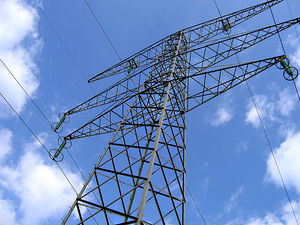# ResistanceFigure 1. High voltage power lines are used for the transmission of electricity over long distances. These are specially designed to have low resistance, so little energy is lost in electrical transmission.

Resistance is similar to friction for electrical energy; resistance causes the electrical energy to be lost as heat (thermal energy), just like friction causes mechanical energy to be lost as heat.

Resistance is a measure of how much voltage an electrical element needs in order to increase the electric current. The actual resistance depends on both the resistivity and the geometry of the wire. Resistivity is a property of the material that helps determine how well it will conduct electricity (metals have low resistivity; wood has high resistivity). A higher resistance results in a lower current for a given voltage. Resistance is measured in Ohms (Ω).

While it is easy to think of resistance as a bad thing, many electronic circuits require resistors (objects with high resistance deliberately added to a circuit) to function!The resistance of a wire increases as its length increases and decreases as its thickness increases. When conducting electricity over long distances, like the electrical grid, it's important to keep the resistance as low as possible. The wires in the electrical grid have a low resistivity and fairly large cross-sectional areas to allow them to conduct electricity over long distances with minimal loss. Please play with the simulation below to see how these values affect resistance.

## PhET: Resistance in a wire

The University of Colorado has graciously allowed us to use the following Phet simulation. Explore the simulation to see how resistance changes depending on geometry and resistivity: